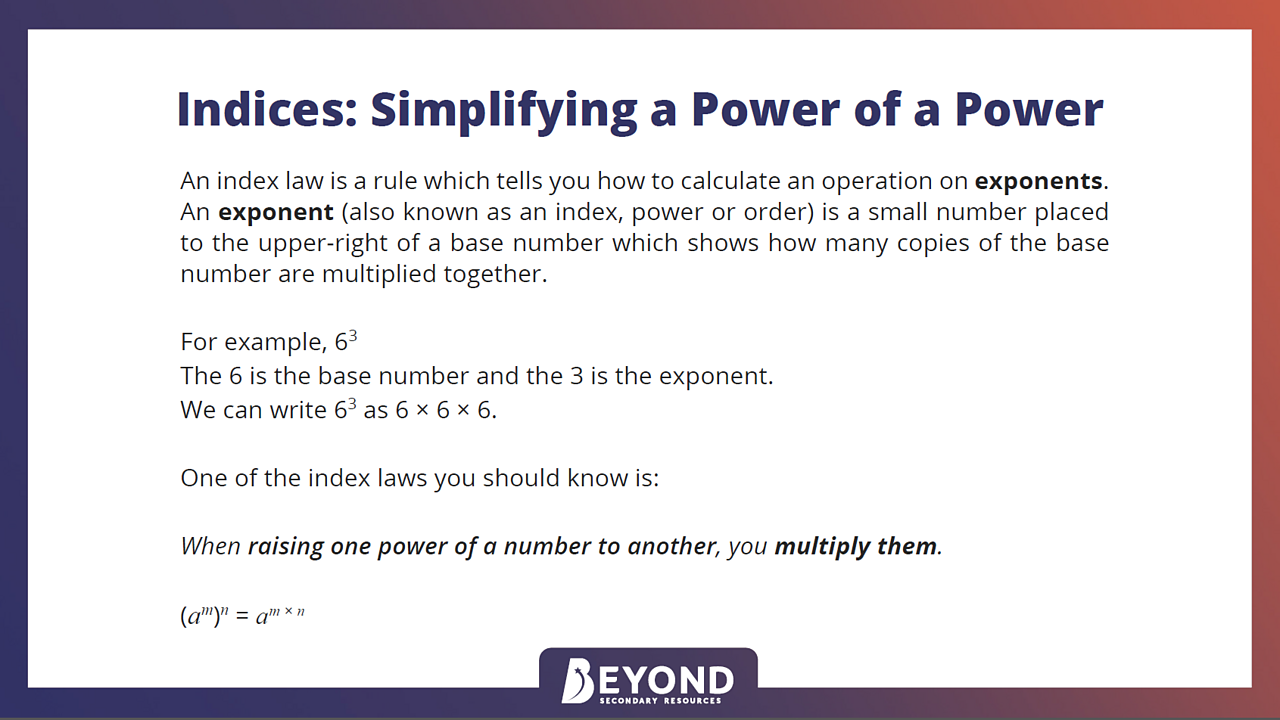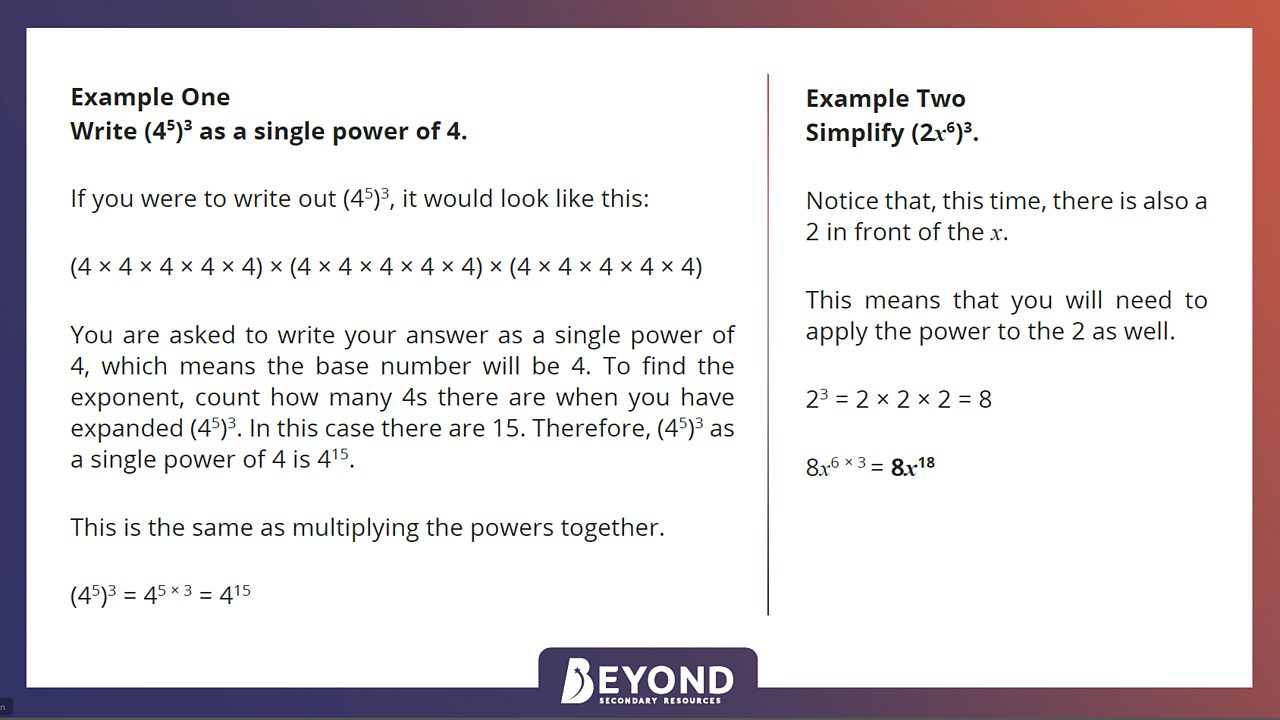# Simplify a power of a power

## Home learning focus

Learn how to simplify a power of a power.

This lesson includes:

• one video
• a learning summary

Created in partnership with Beyond.

# Learn

## Indices

Squares, cubes and higher powers are shown as small digits called indices (the singular form is index), also commonly known as powers or exponents. Indices are used to show numbers that have been multiplied by themselves, and can also be used instead of the roots, such as the square root.

The mathematical laws around indices make complex calculations involving them easier.

### Simplifying a power of a power

You may be asked to simplify a power of a power or raise indices to a power, eg Simplify (aᵐ)ⁿ

If this happens, you are being asked to multiply everything inside the bracket, by itself, the number of times outside the bracket. When raising one power of a number to another, you simply multiply the powers together.

(aᵐ)ⁿ = aᵐ ˣ ⁿ

Follow the slideshow below to learn more about simplifying a power of a power, which is one of the index laws. Read through each example, following the steps closely to see how each solution is arrived at.Index law: Raising one power of a number to anotherTo raise one power of a number to another, simply multiply them.1 of 2Writing amounts as a single power and simplifyingYou could write out the powers then add them all together, or just multiply the indices.2 of 2

For a short further explanation to aid your understanding of raising indices to a power before simplifying, watch the section between 2:44 and 3:11 in this video from BBC Bitesize, National 5 Maths.

# Practise

## Activity 1

Simplifying a power of a power - mixed practice

Now test your understanding and knowledge of raising a power to a power by working through these worksheets from Beyond. Questions are grouped by difficulty either as Bronze, Silver or Gold so aim to get as far as you can! There is also a challenge to complete at the end. Write your answers on a piece of paper.